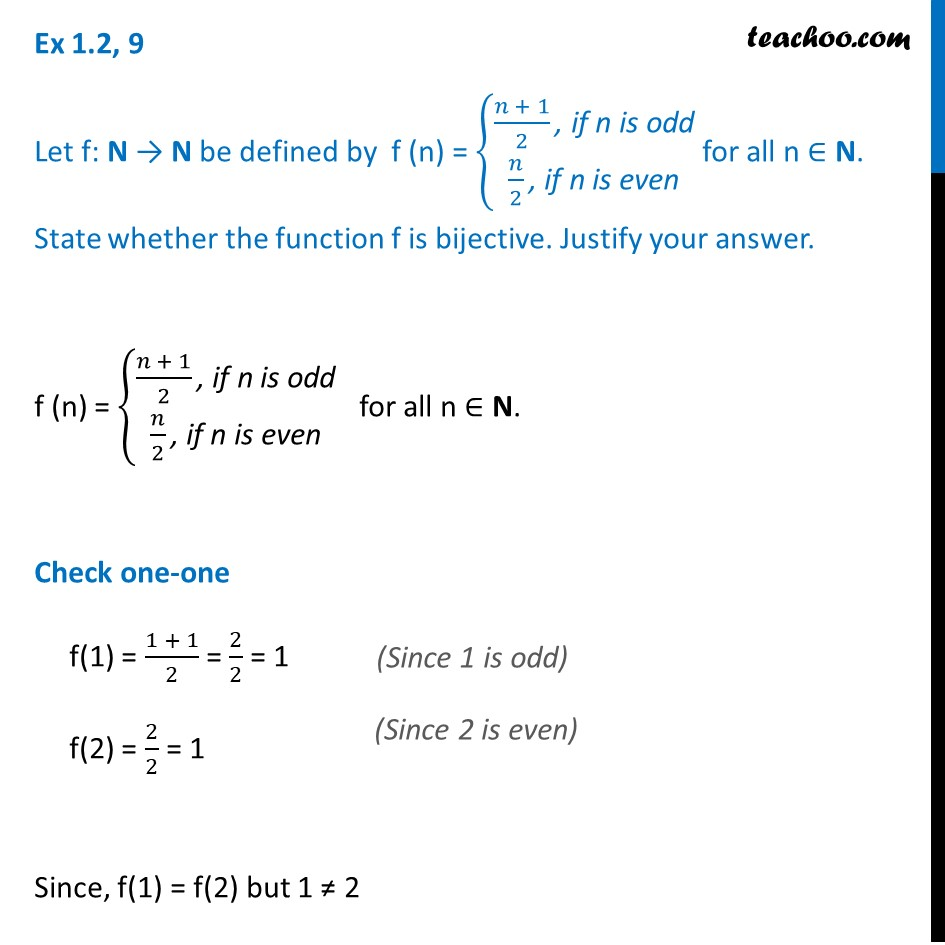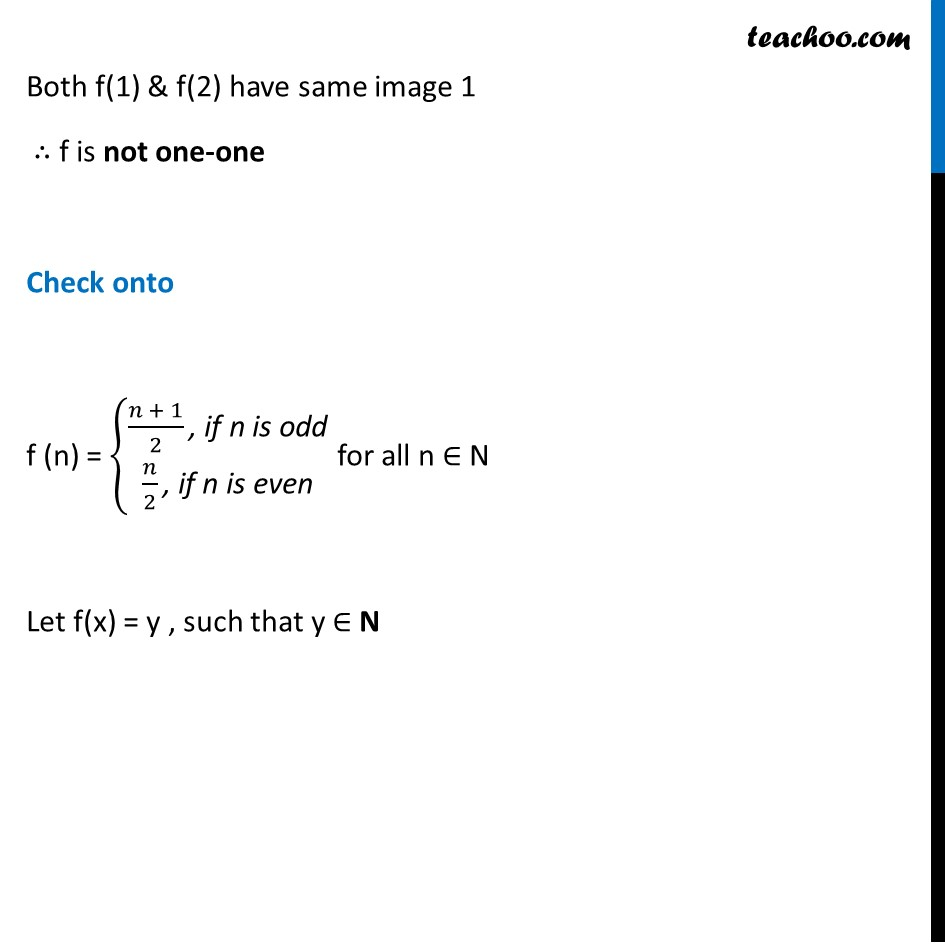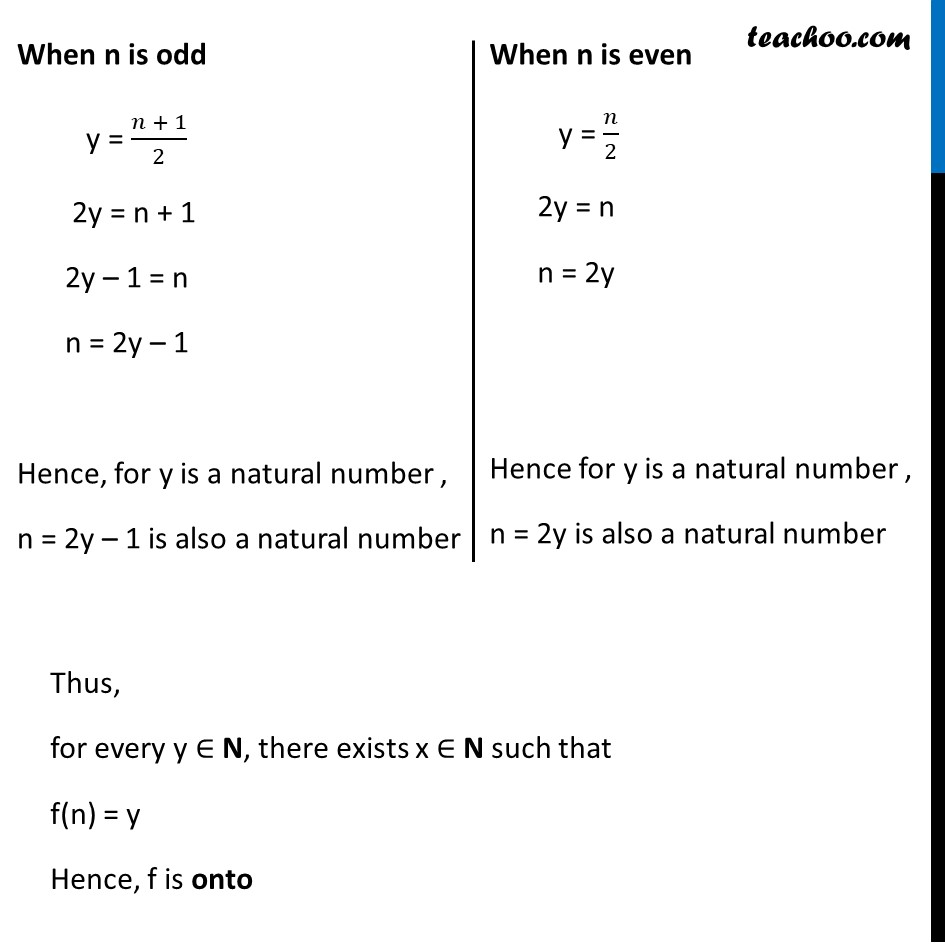Ex 1.2

Chapter 1 Class 12 Relation and Functions
Serial order wiseLearn in your speed, with individual attention - Teachoo Maths 1-on-1 Class

### Transcript

Ex 1.2, 9 Let f: N → N be defined by f (n) = {█((𝑛 + 1)/2 ", if n is odd" @𝑛/2 ", if n is even" )┤ for all n ∈ N. State whether the function f is bijective. Justify your answer. f (n) = {█((𝑛 + 1)/2 ", if n is odd" @𝑛/2 ", if n is even" )┤ for all n ∈ N. Check one-one f(1) = (1 + 1)/2 = 2/2 = 1 f(2) = 2/2 = 1 Since, f(1) = f(2) but 1 ≠ 2 Both f(1) & f(2) have same image 1 ∴ f is not one-one Check onto f (n) = {█((𝑛 + 1)/2 ", if n is odd" @𝑛/2 ", if n is even" )┤ for all n ∈ N Let f(x) = y , such that y ∈ N When n is odd y = (𝑛 + 1)/2 2y = n + 1 2y – 1 = n n = 2y – 1 Hence, for y is a natural number , n = 2y – 1 is also a natural number When n is even y = 𝑛/2 2y = n n = 2y Hence for y is a natural number , n = 2y is also a natural number Thus, for every y ∈ N, there exists x ∈ N such that f(n) = y Hence, f is onto